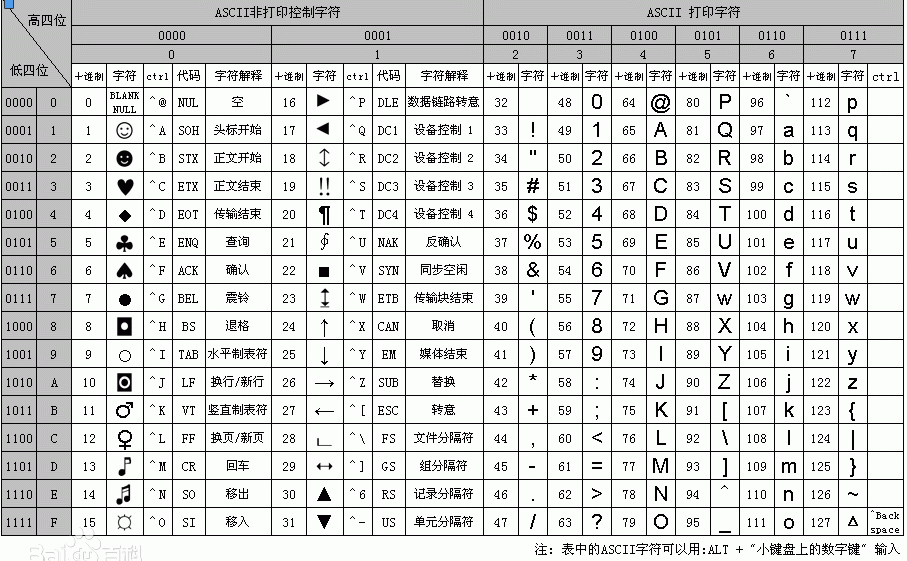# Python中的random函数

## Python中的random函数

random模块提供生成伪随机数的函数，在使用时需要导入random模块

1. random.random()

2.random.uniform(a,b)

3.random.randint(m,n)

4.random.choice(sequence)

5.random.sample(sequence,count)

6.random.randrange([start], stop[, step])

7.random.shuffle(list)

#### 随机生成6位验证码

1. 大写字母、小写字母或数字对应的ASCII表
2. chr()函数
3. 擅长利用random函数``````import random
list5=[]
for i in range(0,2):
list5.append(random.sample([chr(random.randint(65,90)),
chr(random.randint(48,57)),chr(random.randint(97,122))],3))
str5=''.join(list5+list5)
print("生成的验证码为："+str5)
``````

``````import random
list5=[]
for i in range(0,6):
list5.append(random.choice([chr(random.randint(65,90)),
chr(random.randint(48,57)),chr(random.randint(97,122))]))
str5=''.join(list5)
print("生成的验证码为："+str5)
``````